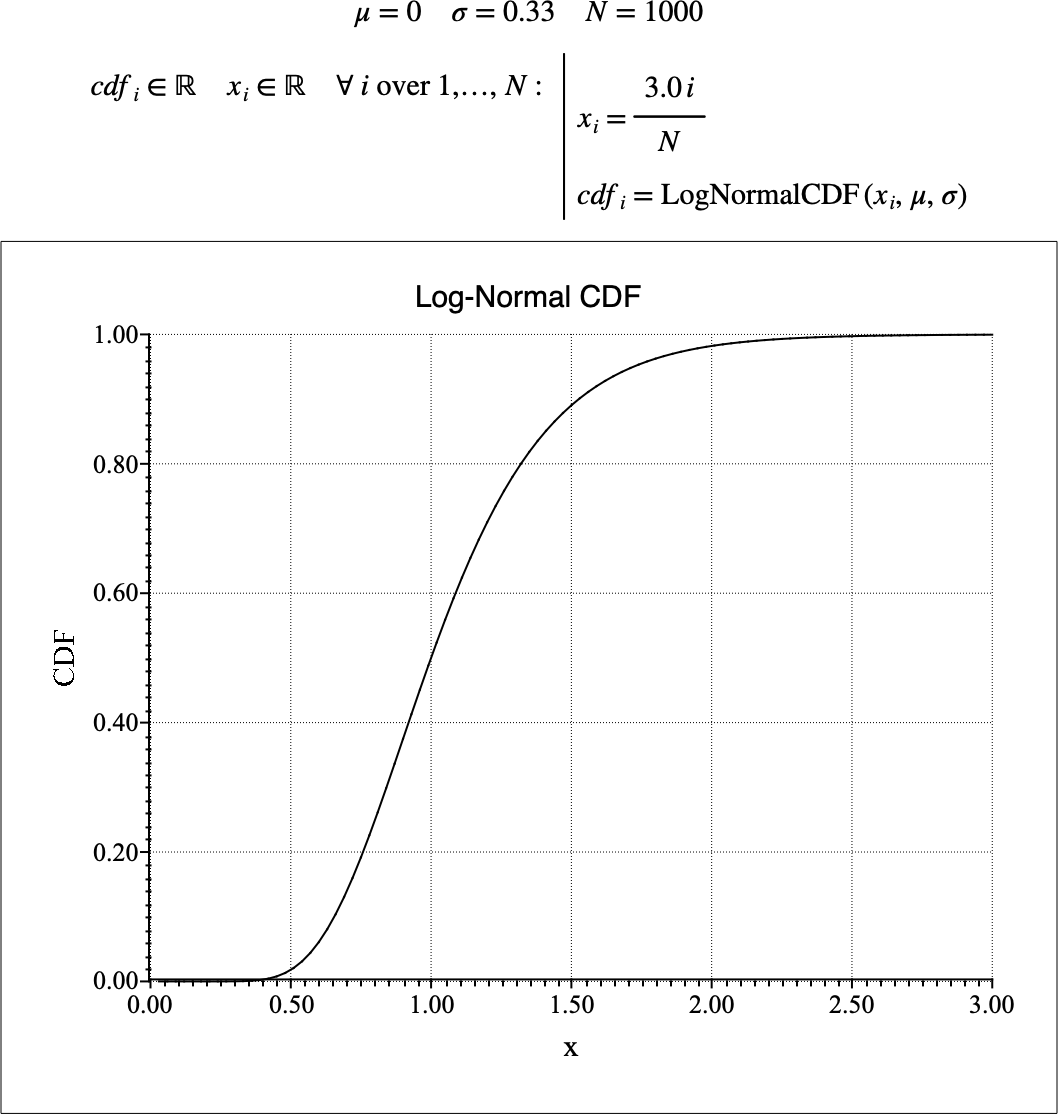# $$\text{LogNormalCDF}$$¶

You can use the $$\text{LogNormalCDF}$$ function to calculate the cumulative distribution function (CDF) of the log-normal distribution.

You can use the \lognormalc backslash command to insert this function.

The following variants of this function are available:

• $$\text{real } \text{LogNormalCDF} \left ( \text{<x>} \right )$$

• $$\text{real } \text{LogNormalCDF} \left ( \text{<x>}, \text{<}\mu\text{>} \right )$$

• $$\text{real } \text{LogNormalCDF} \left ( \text{<x>}, \text{<}\mu\text{>}, \text{<}\sigma\text{>} \right )$$

Where $$x$$, $$\mu$$, and $$\sigma$$ are scalar values representing the value of interest, the mean value and the standard deviation. If not specified, the mean value will be 0 and the standard deviation will be 1. Note that this function is defined over the range $$x > 0$$ and $$\sigma > 0$$. The $$\text{LogNormalCDF}$$ function will generate a runtime error or return NaN for values for which the function is not defined.

The value is calculated directly using the relation:

$\text{LogNormalCDF} \left ( x, \mu, \sigma \right ) = \frac{1}{2} + \frac{1}{2} \text{erf} \left [ \frac{ \ln \left ( x \right ) - \mu }{\sqrt{2} \sigma } \right ]$

Figure 171 shows the basic use of the $$\text{LogNormalCDF}$$ function.Figure 171 Example Use Of the LogNormalCDF Function